×

Data

# A Definitive Guide to Creating Python UDTFs in Snowflake Using Snowpark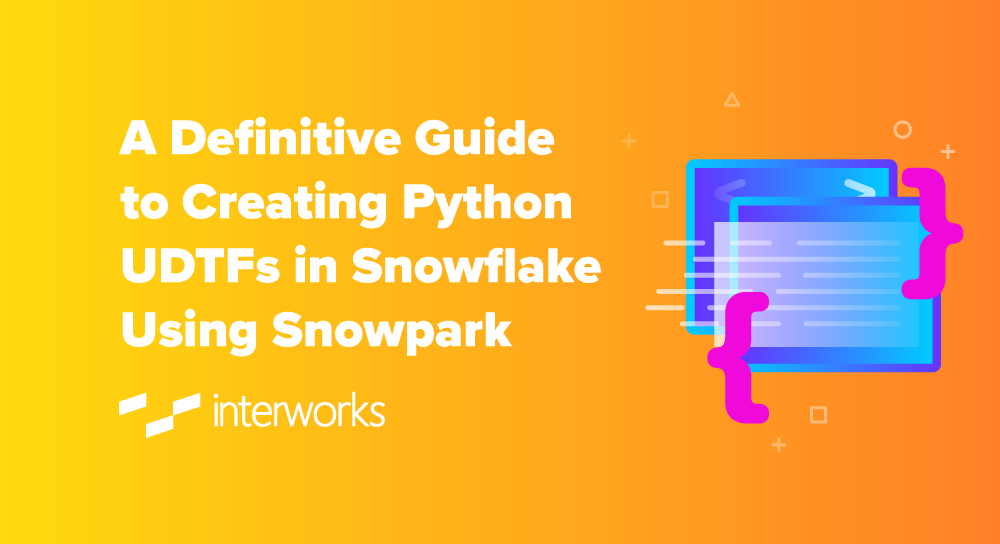This series shows you the various ways you can use Python within Snowflake.

Snowpark for Python is the name for the new Python functionality integration that Snowflake has recently developed. At the Snowflake Summit in June 2022, Snowpark for Python was officially released into Public Preview, which means anybody is able to get started using Python in their own Snowflake environment.

I would understand if you wanted to skip this post and cut directly to the Snowflake’s monolithic documentation page; however, I would strongly recommend reading through this post first, along with my other definitive guides for Python and Snowflake, as these posts will give you an introduction to both the possibilities and limitations of this new functionality whilst walking you through how to get going yourself. We will also walk through the steps you can take to create your own Python User Defined Table Function, or Python UDTF for short, by connecting to Snowflake as part of an external Python script using the Snowpark for Python package.

If you are interested in finding out about Python UDFs or stored procedures instead of UDTFs, or wish to achieve this from within the Snowflake User Interface instead, be sure to check out my other Definitive Guides for Snowflake with Python.

## An Introduction to Python UDTFs in Snowflake

If you haven’t already, I would strongly suggest that you read my previous introductory article in my series of Definitive Guides for Snowflake with Python: An Introduction to Python UDTFs in Snowflake. This article specifically targets the required background around UDTFs that will be relevant for this definitive guide.

## Snowflake Snowpark Sessions

This post focuses on leveraging Snowflake Snowpark Sessions from the Snowpark for Python package to connect to Snowflake and create Python UDTFs. If you haven’t already, I would recommend reading my Definitive Guide to Snowflake Sessions with Snowpark for Python as this will give you a strong introduction into setting up a Snowpark environment to connect to Snowflake and provide you with a few shortcuts in the form of code snippets.

Throughout this blog post, I will always use the variable snowpark_session to refer to a Snowflake Snowpark Session that we have established using the above-mentioned post.

## How to Create Your Own Python UDTF from a Snowflake Snowpark Session

Snowflake have integrated the ability to create Python UDTFs directly into the standard commands that can be executed for a Snowflake Snowpark Session object. Here is a sample Python code to demonstrate how to create your own UDF using Snowpark.

This could easily be overwhelming at this stage so we will follow this template’s description with some examples, starting off simple and getting more complex as we go.

This template includes lines regarding optional packages and imports that will be discussed after our simple examples.

# Define Python class locally
'''
Define main Python class which is
leveraged to process partitions.
Executes in the following order:
- __init__ | Executes once per partition
- process | Executes once per input row within the partition
- end_partition | Executes once per partition
'''
class <name of main Python class> :

'''
Optional __init__ method to
execute logic for a partition
before breaking out into rows
'''
def __init__(self) :
# Python code at the partition level

'''
Method to process each input row
within a partition, returning a
tabular value as tuples.
'''
def process(self, <arguments>) :
'''
Enter Python code here that
executes for each input row.
This likely ends with a set of yield
clauses that output tuples,
for example:
'''
yield (<field_1_value_1>, <field_2_value_1>, ...)
yield (<field_1_value_2>, <field_2_value_2>, ...)
'''
Alternatively, this may end with
a single return clause containing
an iterable of tuples, for example:
'''
return [
(<field_1_value_1>, <field_2_value_1>, ...)
, (<field_1_value_2>, <field_2_value_2>, ...)
]

'''
Optional end_partition method to
execute logic for a partition
after processing all input rows
'''
def end_partition(self) :
# Python code at the partition level
'''
This ends with a set of yield
clauses that output tuples,
for example:
'''
yield (<field_1_value_1>, <field_2_value_1>, ...)
yield (<field_1_value_2>, <field_2_value_2>, ...)
'''
Alternatively, this ends with
a single return clause containing
an iterable of tuples, for example:
'''
return [
(<field_1_value_1>, <field_2_value_1>, ...)
, (<field_1_value_2>, <field_2_value_2>, ...)
]

# Import data types for defining the tabular output structure
from snowflake.snowpark.types import StructType, StructField

# Import data types for specific fields in input and/or output
from snowflake.snowpark.types import <specific Snowpark DataType objects>

# Optional: Import additional packages or files
snowpark_session.add_packages('<list of required packages natively available in Snowflake (i.e. included in Anaconda Snowpark channel)>')

snowpark_session.udtf.register(
handler = <name of main Python class>
, output_schema = StructType(<list of StructField objects with specific field name and Snowpark DataType objects>)
, input_types = <list of input DataType() objects for input parameters>
, is_permanent = True
, name = '<UDTF name>'
, replace = True
, stage_location = '@<UDTF stage name>'
)

The main elements to understand here are:

• On rows 1-67, we define the main Python handler class that will be leveraged by our UDTF. This class can leverage other functions that you have defined in your script or imported from elsewhere, however the UDTF can only be assigned a single main Python handler class.
• On rows 80-88, we leverage the udtf() method of the Snowflake Snowpark Session object to create a new UDTF in Snowflake.
• On row 81, we determine the Python class that will be leveraged by our UDTF. The name here would match the class name on row 10.
• On row 85, we determine the name of the UDTF within Snowflake. You can pass a fully qualified name here if you prefer, otherwise the UDTF will be created in the same namescace as your Snowflake Snowpark Session object.
• On row 87, we determine the name of the Snowflake stage to which the files for our UDTF will be uploaded.
• On row 84, we determine that the UTDF we create will not be temporary. A temporary UDTF will only exist within our specific Snowflake Snowpark Session object.
• On row 86, we determine whether or not to overwrite an existing UDTF with the same name. If this is set to False and a UDTF already exists, an error will be returned.
• On rows 69-73, we import the specific Snowpark DataType objecs that will be leveraged on rows 82-83
• On row 70, we import the specific Snowpark DataType objects that are used to define tabular structures. StructType represents a table schema, whilst StructField represents a field within a table.
• On row 73, we import the specific Snowpark DataType object that will be used for the value passed into the UDTF and the values returned within the StructField‘s output by the UDTF. This is discussed more in a note below.
• On row 82, we define the output schema leveraging the Snowpark DataType objects that were imported on row 73 and embedding them into the StructField and StructType objects imported on row 70.
• On row 83, we define the list of specific Snowpark DataType objects that will be used for the input arguments for the UDTF. All of these must be included in the import on row 73. It is important to note that these Snowpark Datatype objects must cover all expected arguments passed to the process method within the handler class on row 25, and should also be provided in the same order.
• On rows 76 and 77, we provide optional rows to add additional packages and imports to our UDTF. This will be discussed after our simple examples.

### Snowpark DataType Objects

Snowpark must leverage compatible data types for inputs and returned value when creating UDTFs, UDFs and Stored Procedures. You can find the full list of types in Snowflake’s documentation. The examples provided in this blog leverage several different data types, which should give you a reasonable idea for how to configure your own use-cases.

### Simple Examples

Let’s break this down and provide a few simple examples to explain what is happening more clearly.

Please note that these examples are deliberately minimalistic in their design, and thus do not include any error handling or similar concepts. Best practices would be to prepare functions with error handling capabilities to prevent unnecessary execution and provide useful error outputs to the end user.

#### Return a Simple Table

Our first example is a very simple function that returns a static table. First, let’s see the code:

##################################################################
## Define the class for the UDTF

class simple_table :

def process(self) :
'''
Enter Python code here that
executes for each input row.
This ends with a set of yield
clauses that output tuples
'''
yield (1, 'Hello')
yield (2, 'World')

'''
Alternatively, this may end with
a single return clause containing
an iterable of tuples, for example:

return [
(1, 'Hello')
, (2, 'World')
]
'''

##################################################################
## Register UDTF in Snowflake

### Add packages and data types
from snowflake.snowpark.types import StructType, StructField
from snowflake.snowpark.types import IntegerType, StringType

snowpark_session.udtf.register(
handler = simple_table
, output_schema = StructType([StructField("ID", IntegerType()), StructField("NAME", StringType())])
, is_permanent = True
, name = 'SNOWPARK_HELLO_WORLD'
, replace = True
, stage_location = '@UDTF_STAGE'
)

There are a few things to break down here to confirm our understanding:

• Starting on row 4, we have defined a very simple class in Python which acts as our handler. The class only leverages the process method, without using any inputs, and simply returns two tuples of data.
• The name of our handler class on row 4 matches that of our handler class assigned on row 36.
• On row 37, we can see that our function will return a table consisting of an ID integer field and a NAME text field. This output schema leverages the data types imported on rows 31-32, where IntegerType() is used for integers and StringType() is used for floats.

If we execute this code as part of a Python script, we can then call the table function using the snowpark_session.sql().show() method to see the result.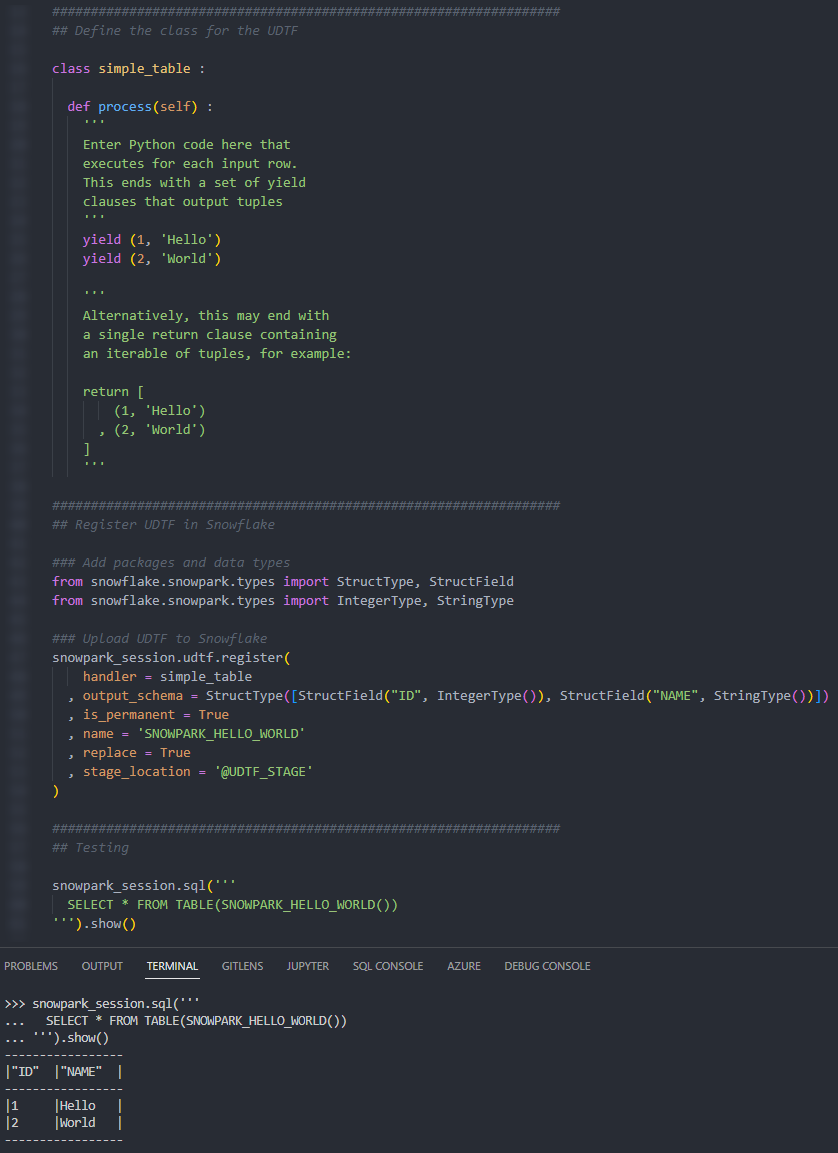We can also call the table function from within a Snowflake worksheet to see the result.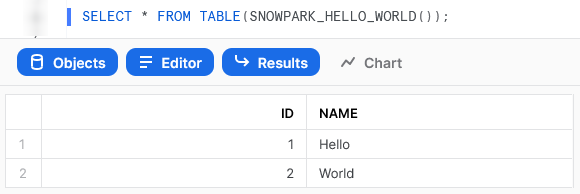#### Return a Simple Table Based on an Input

Our second example is a step up from the first, following a similar process but using an input variable to determine the output. Let’s see the code:

##################################################################
## Define the class for the UDTF

class simple_table_from_input :

def process(self, row_count: int) :

# Leverage an if statement to output
# a different result depending on the input
if row_count is None or row_count not in [1, 2] :
return None
elif row_count == 1 :
return [(1, 'Hello World')]
elif row_count == 2 :
return [
(1, 'Hello')
, (2, 'World')
]

##################################################################
## Register UDTF in Snowflake

### Add packages and data types
from snowflake.snowpark.types import StructType, StructField
from snowflake.snowpark.types import IntegerType, StringType

snowpark_session.udtf.register(
handler = simple_table_from_input
, output_schema = StructType([StructField("ID", IntegerType()), StructField("NAME", StringType())])
, input_types = [IntegerType()]
, is_permanent = True
, name = 'SNOWPARK_HELLO_WORLD_WITH_INPUT'
, replace = True
, stage_location = '@UDTF_STAGE'
)

This example steps up in difficulty from the first, introducing the following key concepts:

• On row 6, we can see an expected input arguments for the process method in the handler class. This is matched by providing the input types list on row 31.
• On rows 10-18, we leverage an if statement to determine a different result depending on the input.
• For our outputs, we leverage return instead of yield so that we can output the full set of records at once.
• Importantly, note that each potential output still matches the same table structure consisting of an ID integer field and a NAME text field.

Again, if we execute this code as part of a Python script, we can then call the table function using the snowpark_session.sql().show() method to see the result. This screenshot omits the code itself for brevity.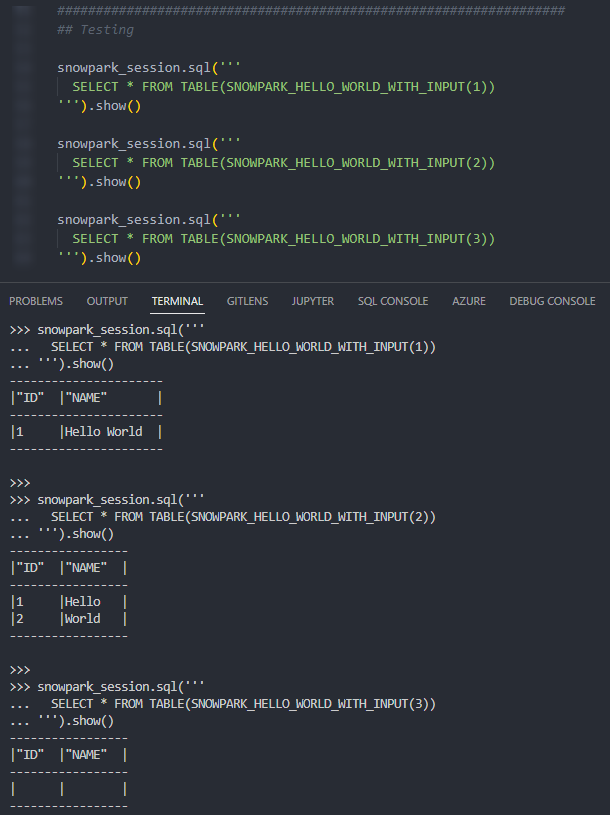Again, we can also call the table function from within a Snowflake worksheet to see the result.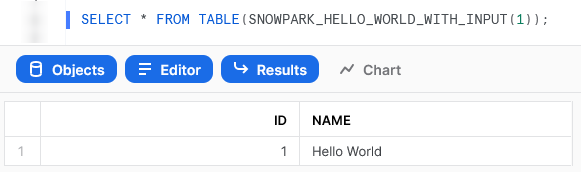#### Calendar Table

The last of our simple examples takes things one step further, so that we can introduce an additional Python function in our script and demonstrate the power of list comprehension. This UDTF leverages two input dates and generates a calendar table.

Let’s see the code:

##################################################################
## Define the class for the UDTF

# Import required standard library
from datetime import date, timedelta

# Define the class for the UDTF
class calendar_table :

def process(
self
, start_date: date
, end_date: date
) :

# Leverage list comprehension to generate dates between start and end
list_of_dates = [start_date+timedelta(days=x) for x in range((end_date-start_date).days + 1)]

# Leverage list comprehension to create tuples of desired date details from list of dates
list_of_date_tuples = [
(
date
, date.year
, date.month
, date.strftime("%B")
, date.strftime("%b")
, date.day
, date.strftime("%A")
, date.strftime("%a")
, date.strftime("%w")
, date.strftime("%j")
, date.strftime("%W")
, date.strftime("%G")
, date.strftime("%V")
, date.strftime("%u")
)
for date in list_of_dates
]

return list_of_date_tuples

##################################################################
## Register UDTF in Snowflake

### Add packages and data types
from snowflake.snowpark.types import StructType, StructField
from snowflake.snowpark.types import DateType, IntegerType, StringType

### Define output schema
output_schema = StructType([
StructField("DATE", DateType())
, StructField("YEAR", IntegerType())
, StructField("MONTH", IntegerType())
, StructField("MONTH_NAME", StringType())
, StructField("MONTH_NAME_SHORT", StringType())
, StructField("DAY", IntegerType())
, StructField("DAY_NAME", StringType())
, StructField("DAY_NAME_SHORT", StringType())
, StructField("DAY_OF_WEEK", IntegerType())
, StructField("DAY_OF_YEAR", IntegerType())
, StructField("WEEK_OF_YEAR", IntegerType())
, StructField("ISO_YEAR", IntegerType())
, StructField("ISO_WEEK", IntegerType())
, StructField("ISO_DAY", IntegerType())
])

snowpark_session.udtf.register(
handler = calendar_table
, output_schema = output_schema
, input_types = [DateType(), DateType()]
, is_permanent = True
, name = 'SNOWPARK_CALENDAR_TABLE'
, replace = True
, stage_location = '@UDTF_STAGE'
)

This example steps up in difficulty in several ways, introducing the following key concepts:

• On rows 12-13, we can see two expected input arguments for the process method in the handler class, which denote the start and end dates for the returned calendar table.
• On rows 50-65, we can see that the metadata for the returned table contains several fields, including the date, year, month and day. We have defined this as a separate variable which is then passed into the udtf.register() method on row 70, as this makes our code easier to read. We will continue this approach for the remaining examples in this article.
• On row 5, we import our required functionality from the standard Python library. As this is from the standard Python library, no further imports or configurations are required.
• On row 17, we leverage list comprehension to generate a list of dates between the provided start and end dates.
• On rows 20-38, we again leverage list comprehension to create a list of tuples that contain the desired details for each date in the list of dates.
• On row 40, we leverage return instead of yield so that we can output the full set of records at once. It is critical that the output being returned is an iterable of tuples.

Again, if we execute this code as part of a Python script, we can then call the table function using the snowpark_session.sql().show() method to see the result. This screenshot omits the code itself for brevity.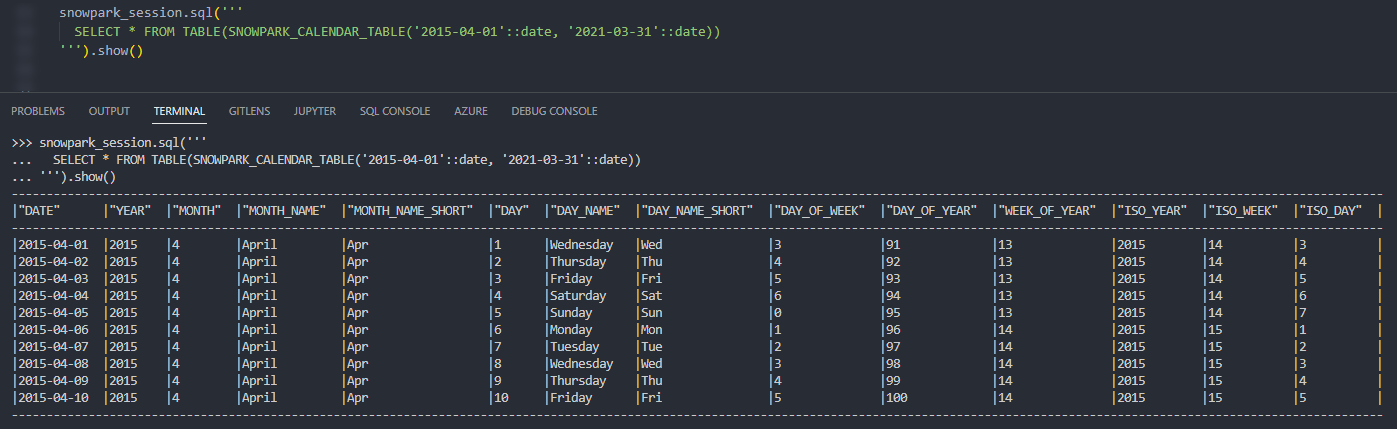Again, we can also call the table function from within a Snowflake worksheet to see the result.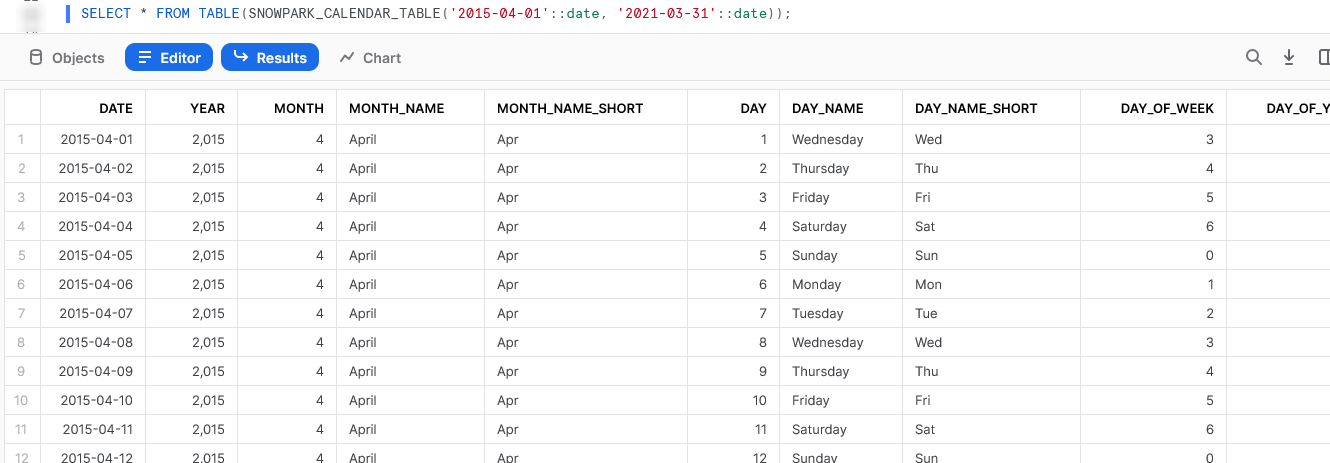### Examples Leveraging Partitioned Data

Now that we have some simple examples under our belt, it’s time to progress to some examples leveraging input data. In my opinion, this is where UDTFs really start to shine and demonstrate their potential.

#### Running Sum

This is a simple example that demonstrates using partitioning within a UDTF to act on a tabular input. Of course, we could easily achieve the same result using a standard window function in SQL, but we are trying to demonstrate functionality here and this is a simple example.

Let’s see the code:

##################################################################
## Define the class for the UDTF

# Define handler class
class generate_running_sum :

## Define __init__ method that acts
## on full partition before rows are processed
def __init__(self) :
# Create initial running sum variable at zero
self._running_sum = 0

## Define process method that acts
## on each individual input row
def process(
self
, input_measure: float
) :

# Increment running sum with data
# from the input row
new_running_sum = self._running_sum + input_measure
self._running_sum = new_running_sum

yield(new_running_sum,)

##################################################################
## Register UDTF in Snowflake

### Add packages and data types
from snowflake.snowpark.types import StructType, StructField
from snowflake.snowpark.types import FloatType

### Define output schema
output_schema = StructType([
StructField("RUNNING_SUM", FloatType())
])

snowpark_session.udtf.register(
handler = generate_running_sum
, output_schema = output_schema
, input_types = [FloatType()]
, is_permanent = True
, name = 'SNOWPARK_GENERATE_RUNNING_SUM'
, replace = True
, stage_location = '@UDTF_STAGE'
)

The core difference between this UDTF and our previous examples are:

• On rows 9-11, we have now included the __init__ method within our class. We use this method to declare a variable called _running_sum within self that begins with zero value.
• On row 22, we leverage the self._running_sum variable to calculate the new running sum for the current row. If this is the first row, the self._running_sum value will be zero as we initialised on row 11. If this is not the first row, it will use the previous row’s final value of self._running_sum which we set on row 23.
• It would be tempting to yield self._running_sum itself on row 25 instead of an interim variable such as new_running_sum, however this would be reading the value from the original input instead of the updated value, and thus would not include any of the change we make. If you attempt this yourself, you will find it returns the same as the input instead of a running total.
• Our yield statement on row 25 looks slightly strange due to the comma near the end. We must leverage this comma to ensure that our single-value output is treated as a tuple instead of a scalar variable.

When calling the UDTF using values from another query as an input, we leverage the following SQL statement. This statement queries a table called DEMO_SALES_DATA and uses comma notation to indicate leveraging a tabular UDTF. This is similar to when performing a lateral flatten to parse semi-structured data. We must also strictly determine which fields we wish to partition our data by and the order in which rows should be submitted, similar to how we would for a window function.

select *
from DEMO_SALES_DATA
, table(
SNOWPARK_GENERATE_RUNNING_SUM(SALES)
over (
partition by CATEGORY, SUBCATEGORY
order by SALE_DATE asc
)
)
;

Again, if we execute this code as part of a Python script, we can then call the table function using the snowpark_session.sql().show() method to see the result. This screenshot omits the code itself for brevity.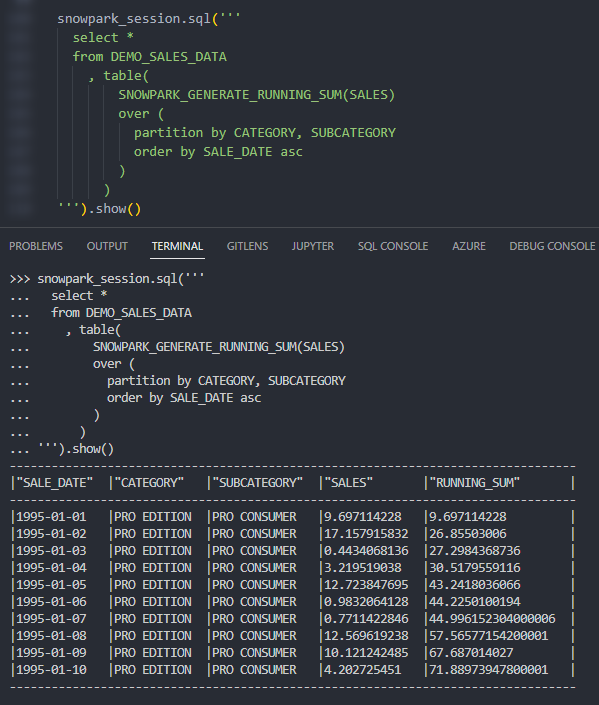Again, we can also call the table function from within a Snowflake worksheet whilst specifying our desired partitions to see the result.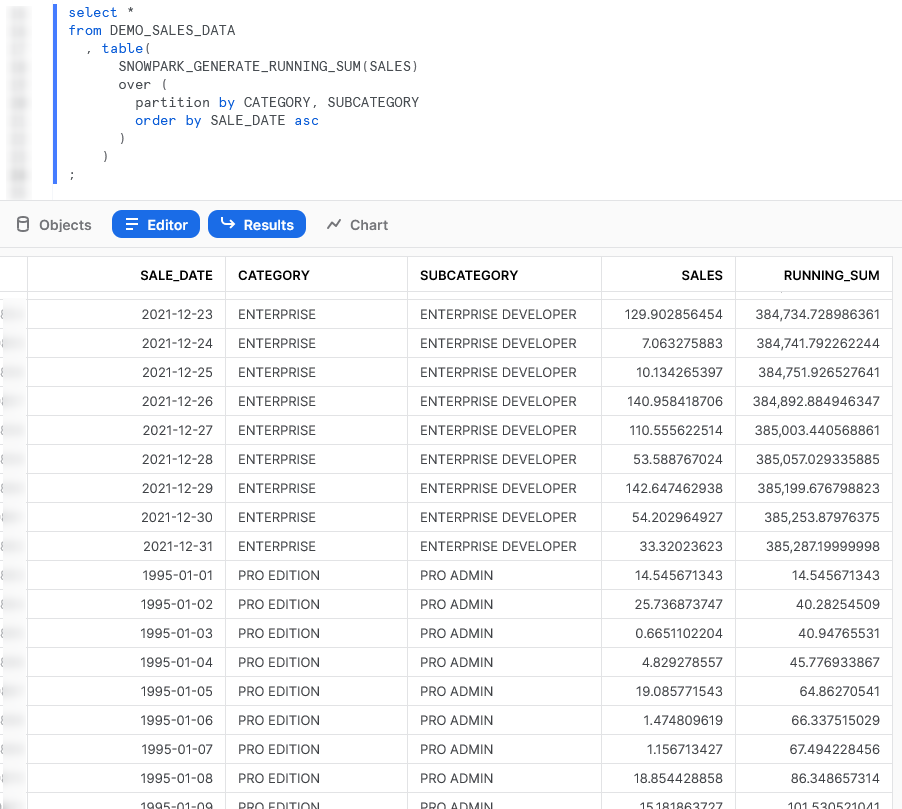#### Average

Our next example again takes things one step further, but is not an efficient or particular useful UDTF. We purely include this to demonstrate the functionality before diving into our more complex examples below. In this example, we read in all of the values for the partition first, then use those values to return the average value for each partition. This would be easily achieved using a simple GROUP BY function in SQL paired with the AVG function.

Let’s see the code:

##################################################################
## Define the class for the UDTF

# Define handler class
class generate_average :

## Define __init__ method that acts
## on full partition before rows are processed
def __init__(self) :
# Create initial empty list to store values
self._values = []

## Define process method that acts
## on each individual input row
def process(
self
, input_measure: float
) :

# Increment running sum with data
# from the input row
self._values.append(input_measure)

## Define end_partition method that acts
## on full partition after rows are processed
def end_partition(self) :
values_list = self._values

average = sum(values_list) / len(values_list)

yield(average,)

##################################################################
## Register UDTF in Snowflake

### Add packages and data types
from snowflake.snowpark.types import StructType, StructField
from snowflake.snowpark.types import FloatType

### Define output schema
output_schema = StructType([
StructField("AVERAGE", FloatType())
])

snowpark_session.udtf.register(
handler = generate_average
, output_schema = output_schema
, input_types = [FloatType()]
, is_permanent = True
, name = 'SNOWPARK_GENERATE_AVERAGE'
, replace = True
, stage_location = '@UDTF_STAGE'
)

The core difference between this UDTF and our previous example is the inclusion of the end_partition method on rows 26-31 which acts on the full list of input values after they have been collected by the process method.

Again, if we execute this code as part of a Python script, we can then call the table function using the snowpark_session.sql().show() method to see the result. This screenshot omits the code itself for brevity.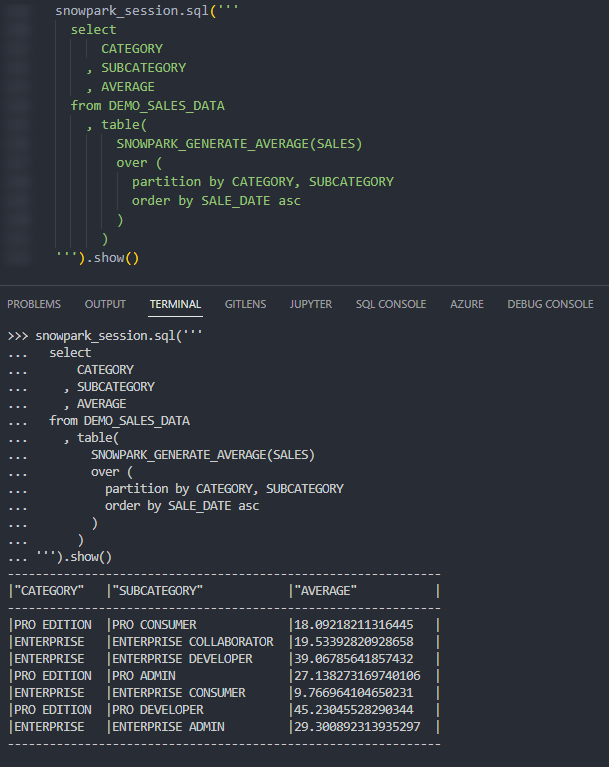Again, we can also call the table function from within a Snowflake worksheet whilst specifying our desired partitions to see the result.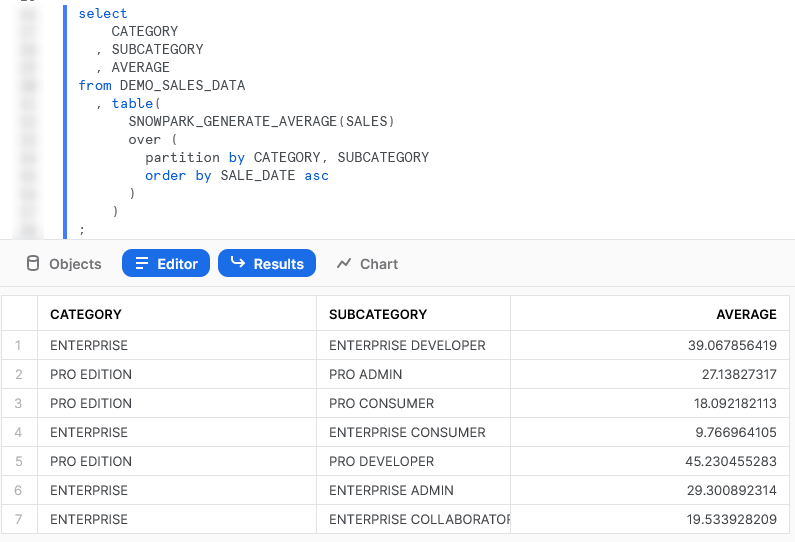## Importing Additional Libraries from Anaconda

Even though our examples so far have all been fairly basic, we can already start to see how powerful Python UDTFs could be. Not only are we able to receive inputs and use them to produce outputs, we can also define our own functionality within the Python script and perform more complex logical steps when executing our function.

What about if we wish to use Python libraries that are not part of the standard inbuilt set? For example, what if we wish to leverage Pandas, PyTorch, or a wide range of other popular libraries? The good news here is that Snowflake have partnered with Anaconda and you already have everything you need to leverage any of the libraries listed in Anaconda’s Snowflake channel.

If you wish to leverage any libraries that are not included in Anaconda’s Snowflake channel, including any libraries you have developed in-house, then you will need to import them separately. This will be discussed in the next section.

### Accepting the Terms of Usage to Enable Third-Party Packages

To leverage third party packages from Anaconda within Snowflake, an ORGADMIN must first accept the third-party terms of usage. I walk through the process now, however more details can be found here for those who desire it. This step must only be completed once for the entire organisation.

1. Using the ORGADMIN role in the SnowSight UI, navigate to Admin > Billing to accept the third party terms of usage.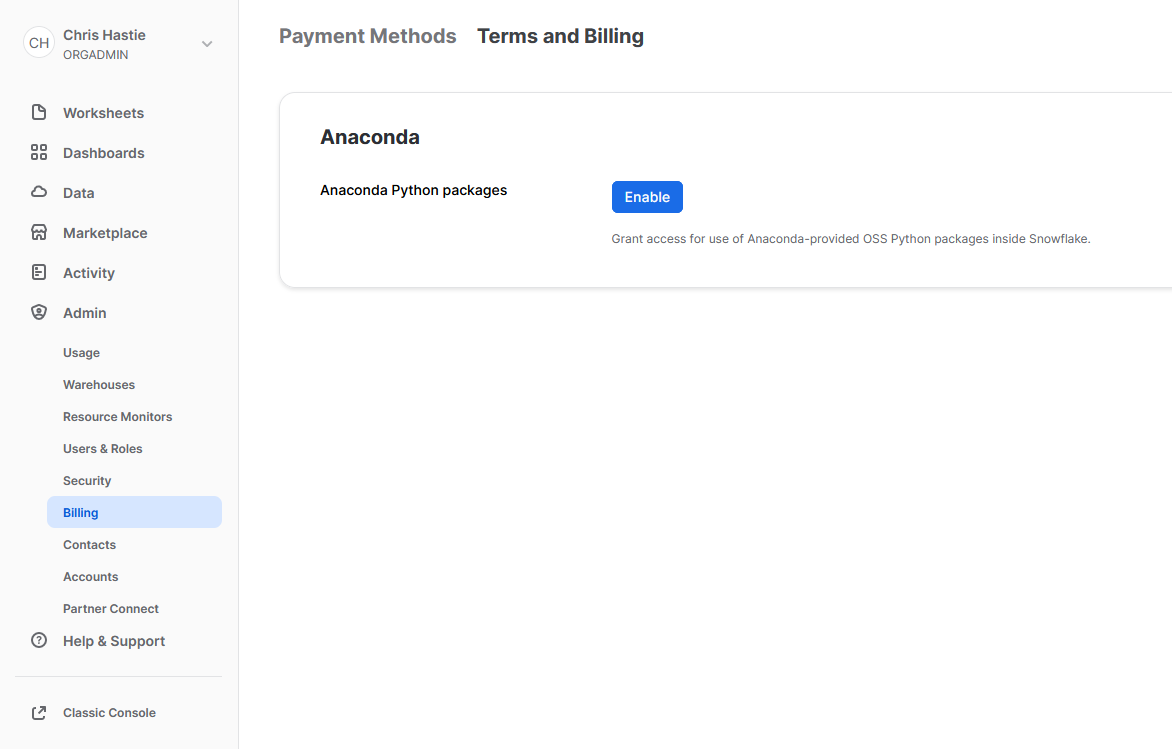2. Confirm acknowledgement.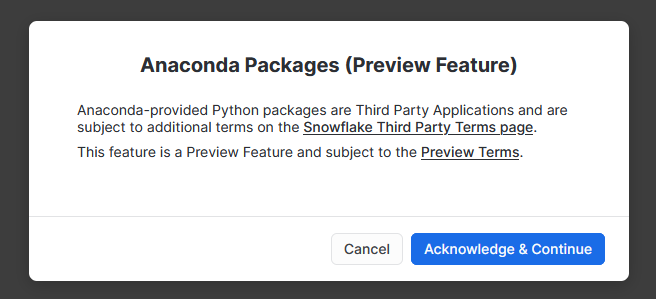3. The screen will then update to reflect the accepted terms.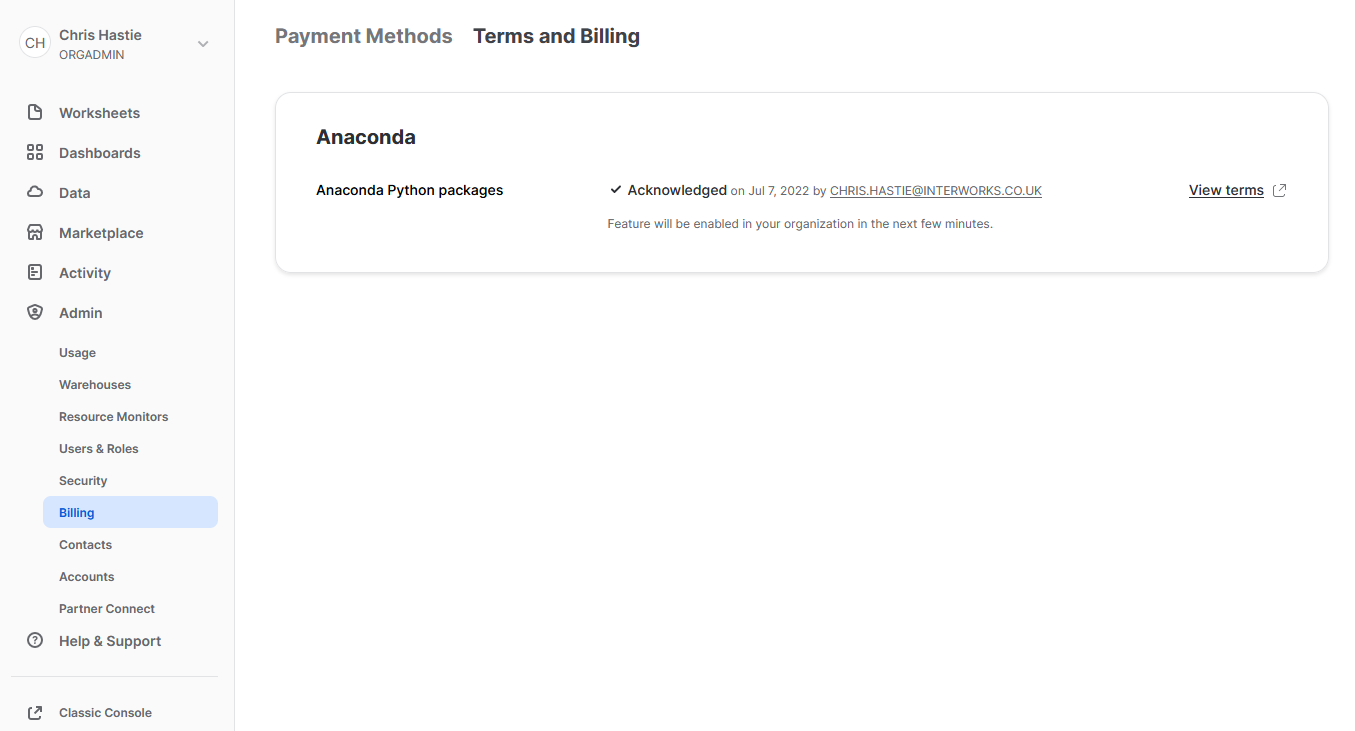### Examples Using Supported Third-Party Libraries

Now that we have enabled third-party libraries for our organisation, we can show some more interesting examples.

#### Train and Deploy an ARIMA Machine Learning Model

This function imports the pmdarima library to train an ARIMA model and then test it, using an 80/20 train/test split. This is useful to demonstrate some of the powerful functionality that is unlocked when you combine Python and Snowflake.

It is important to note at this time that I am not a data scientist by trade and am simply demonstrating functionality; however, I am open to any feedback or knowledge about how this demonstration could be improved. You can also learn more about this approach by attending the session that Thoughtspot’s Sonny Rivera and myself are running at this year’s Snowflake BUILD 2022 Data Cloud Dev Summit.

Let’s see the code:

##################################################################
## Define the class for the UDTF

# Import modules
import pandas
import pmdarima
from datetime import date

# Define handler class
class generate_auto_arima_predictions :

## Define __init__ method that acts
## on full partition before rows are processed
def __init__(self) :
# Create empty list to store inputs
self._data = []

## Define process method that acts
## on each individual input row
def process(
self
, input_date: date
, input_measure: float
) :

# Ingest rows into pandas DataFrame
data = [input_date, input_measure]
self._data.append(data)

## Define end_partition method that acts
## on full partition after rows are processed
def end_partition(self) :

# Convert inputs to DataFrame
df_input = pandas.DataFrame(data=self._data, columns=["DATE", "MEASURE"])

# Determine test and train splits of the data
# leverage an 80:20 ratio.
train_data, test_data = pmdarima.model_selection.train_test_split(df_input["MEASURE"], test_size=0.2)

# Create Auto Arima model
model = pmdarima.auto_arima(
train_data
, max_p=3, max_d=3, max_q=3
, seasonal=True, m=12, max_P=3
, max_D=2, max_Q=3, trace=True
, error_action='ignore'
, suppress_warnings=True
, stepwise=True
)

# Convert train and test values to dataframes
df_train = pandas.DataFrame(data=train_data, columns=["MEASURE_TRAIN"])
df_test = pandas.DataFrame(data=test_data, columns=["MEASURE_TEST"])

# Generate in-sample predictions
pred_train = model.predict_in_sample(dynamic=False) # works only with auto-arima
df_train = pandas.DataFrame(
data=pandas.to_numeric(pred_train)
, columns=["PREDICTION_TRAIN"]
)

# Generate predictions on test data
pred_test = model.predict(n_periods=len(test_data), dynamic=False)
df_test = pandas.DataFrame(
data=pandas.to_numeric(pred_test)
, columns=["PREDICTION_TEST"]
)
# Adjust test index to align with
# the end of the training data
df_test.index += len(df_train)

# Combine test and train prediction values with original
df_output = pandas.concat([df_input, df_train, df_test], axis = 1) \
[["DATE", "MEASURE", "PREDICTION_TRAIN", "PREDICTION_TEST"]]

# Output the result
return list(df_output.itertuples(index=False, name=None))

'''
Alternatively, the output could be returned
by iterating through the rows and yielding them
for index, row in df_output.iterrows() :
yield(row, row, row, row)
'''

##################################################################
## Register UDTF in Snowflake

### Add packages and data types
from snowflake.snowpark.types import StructType, StructField
from snowflake.snowpark.types import DateType, FloatType

### Define output schema
output_schema = StructType([
StructField("DATE", DateType())
, StructField("MEASURE", FloatType())
, StructField("PREDICTION_TRAIN", FloatType())
, StructField("PREDICTION_TEST", FloatType())
])

snowpark_session.udtf.register(
handler = generate_auto_arima_predictions
, output_schema = output_schema
, input_types = [DateType(), FloatType()]
, is_permanent = True
, name = 'SNOWPARK_GENERATE_AUTO_ARIMA_PREDICTIONS'
, replace = True
, stage_location = '@UDTF_STAGE'
)

The core difference between this UDTF and our previous examples are:

• On row 94, we have now included a line to include the pandas and pmdarima libraries in the session being used to create the UDTF.
• On rows 5-6, we import the pandas and pmdarima libraries for use in our Python code.

Again, if we execute this code as part of a Python script, we can then call the table function using the snowpark_session.sql().show() method to see the result. This screenshot omits the code itself for brevity.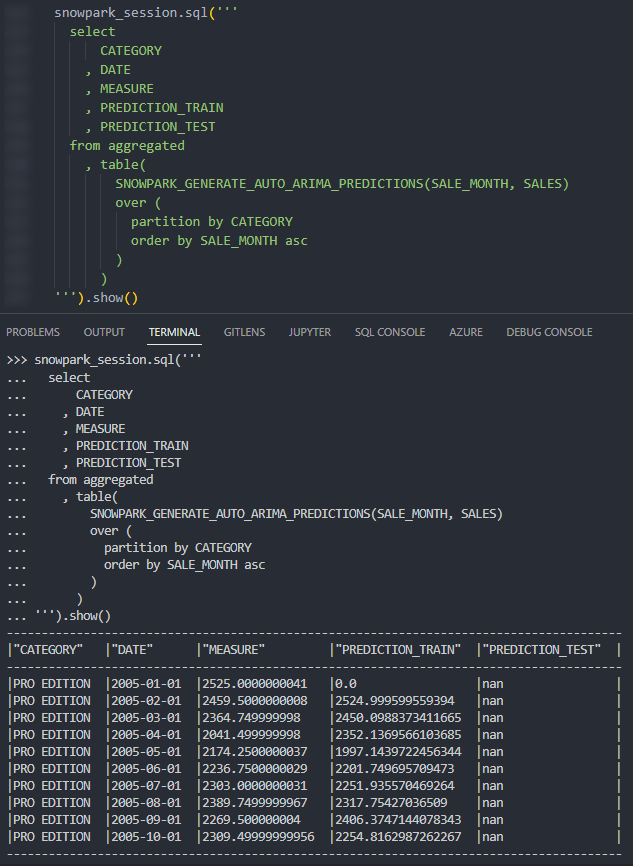Again, we can also call the table function from within a Snowflake worksheet whilst specifying our desired partitions to see the result. Here we display the chart from Snowsight instead of the tabular output so that we can clearly demonstrate how our test predictions compare with our original values.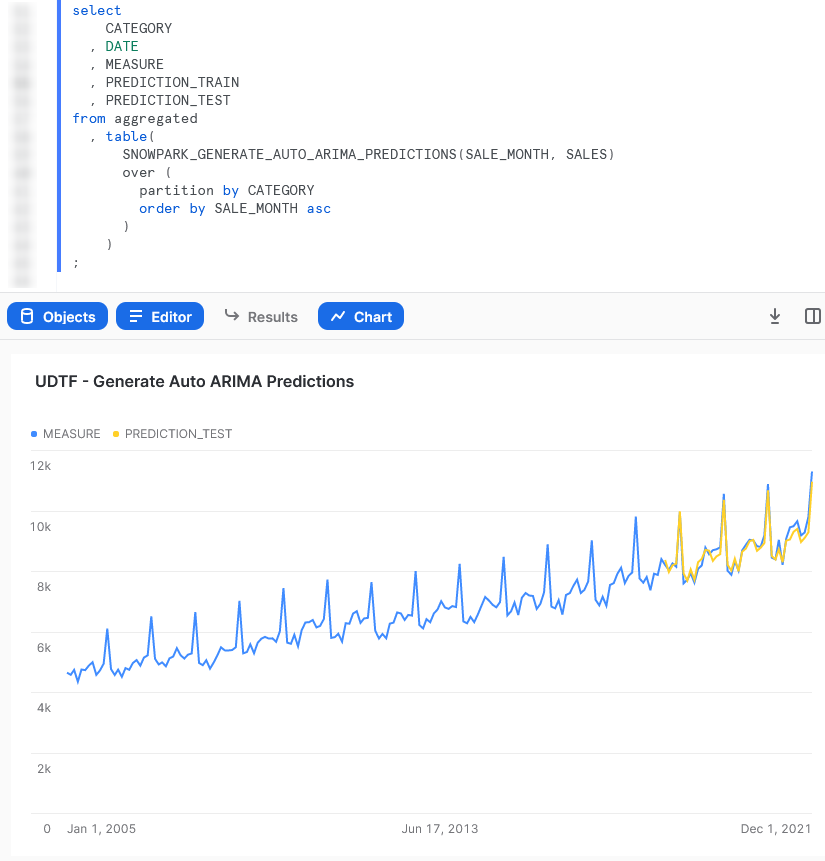#### Train and Deploy a scikit elarn Linear Regression Model

This function imports the scikit learn library to train a linear regression model and then use it to generate a handful of predicted values.

Again, it is important to note at this time that I am not a data scientist by trade and am simply demonstrating functionality; however, I am open to any feedback or knowledge about how this demonstration could be improved.

Let’s see the code:

##################################################################
## Define the class for the UDTF

# Import modules
import pandas
from sklearn.linear_model import LinearRegression
from datetime import date

# Define handler class
class generate_linear_regression_predictions :

## Define __init__ method that acts
## on full partition before rows are processed
def __init__(self) :
# Create empty list to store inputs
self._data = []

## Define process method that acts
## on each individual input row
def process(
self
, input_year: date
, input_measure: float
, input_periods_to_forecast: int
) :

# Ingest rows into pandas DataFrame
data = [input_year, 'ACTUAL', input_measure]
self._data.append(data)
self._periods_to_forecast = input_periods_to_forecast

## Define end_partition method that acts
## on full partition after rows are processed
def end_partition(self) :

# Convert inputs to DataFrame
df_input = pandas.DataFrame(data=self._data, columns=["YEAR", "TYPE", "MEASURE"])

# Determine inputs for linear regression model
# x = pandas.DatetimeIndex(df_input["DATE"]).year.to_numpy().reshape(-1, 1)
x = df_input["YEAR"].to_numpy().reshape(-1, 1)
y = df_input["MEASURE"].to_numpy()

# Create linear regression model
model = LinearRegression().fit(x, y)

# Determine forecast range
periods_to_forecast = self._periods_to_forecast
# Leverage list comprehension to generate desired years
list_of_years_to_predict = [df_input["YEAR"].iloc[-1] + x + 1 for x in range(periods_to_forecast)]

prediction_input = [[x] for x in list_of_years_to_predict]

# Generate predictions and create a df
predicted_values = model.predict(prediction_input)
predicted_values_formatted = pandas.to_numeric(predicted_values).round(2).astype(float)
df_predictions = pandas.DataFrame(
data=predicted_values_formatted
, columns=["MEASURE"]
)

# Create df for prediction year
df_prediction_years = pandas.DataFrame(
data=list_of_years_to_predict
, columns=["YEAR"]
)

# Create df for prediction type
prediction_type_list = ["PREDICTION" for x in list_of_years_to_predict]
df_prediction_type = pandas.DataFrame(
data=prediction_type_list
, columns=["TYPE"]
)

# Combine predicted dfs into single df
df_predictions_combined = pandas.concat([df_prediction_years, df_prediction_type, df_predictions], axis = 1) \
[["YEAR", "TYPE", "MEASURE"]]

# Adjust test index to align with
# the end of the training data
df_predictions.index += len(df_input)

# Combine predicted values with original
df_output = pandas.concat([df_input, df_predictions_combined], axis = 0) \
[["YEAR", "TYPE", "MEASURE"]]

# Output the result
return list(df_output.itertuples(index=False, name=None))

'''
Alternatively, the output could be returned
by iterating through the rows and yielding them
for index, row in df_output.iterrows() :
yield(row, row, row)
'''

##################################################################
## Register UDTF in Snowflake

### Add packages and data types
from snowflake.snowpark.types import StructType, StructField
from snowflake.snowpark.types import IntegerType, StringType, FloatType

### Define output schema
output_schema = StructType([
StructField("YEAR", IntegerType())
, StructField("TYPE", StringType())
, StructField("MEASURE", FloatType())
])

snowpark_session.udtf.register(
handler = generate_linear_regression_predictions
, output_schema = output_schema
, input_types = [IntegerType(), FloatType(), IntegerType()]
, is_permanent = True
, name = 'SNOWPARK_GENERATE_LINEAR_REGRESSION_PREDICTIONS'
, replace = True
, stage_location = '@UDTF_STAGE'
)

This example is very similar to the Auto ARIMA example and simply serves as another opportunity to demonstrate the functionality here.

Again, if we execute this code as part of a Python script, we can then call the table function using the snowpark_session.sql().show() method to see the result. This screenshot omits the code itself for brevity.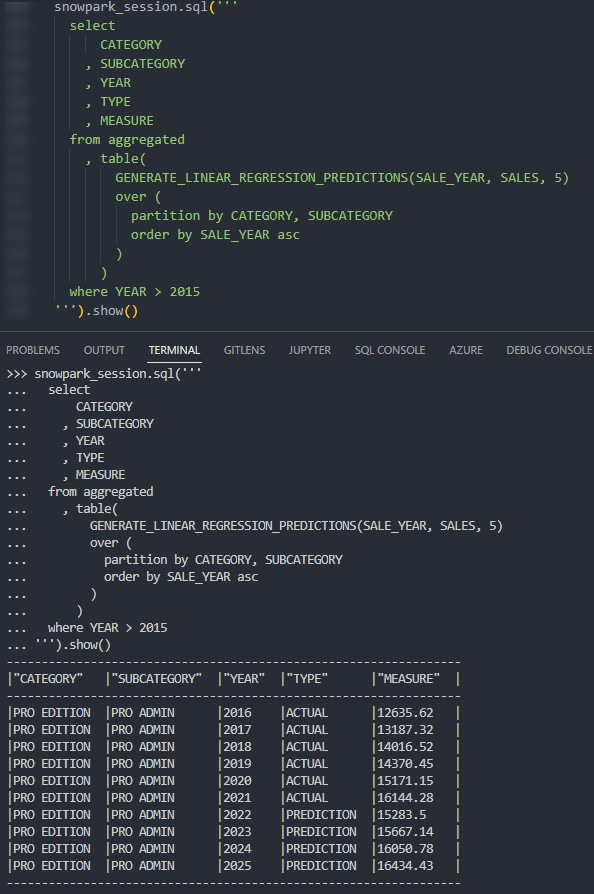Again, we can also call the table function from within a Snowflake worksheet whilst specifying our desired partitions to see the result. Here we can see five predicted values along with the final actual values for the partition.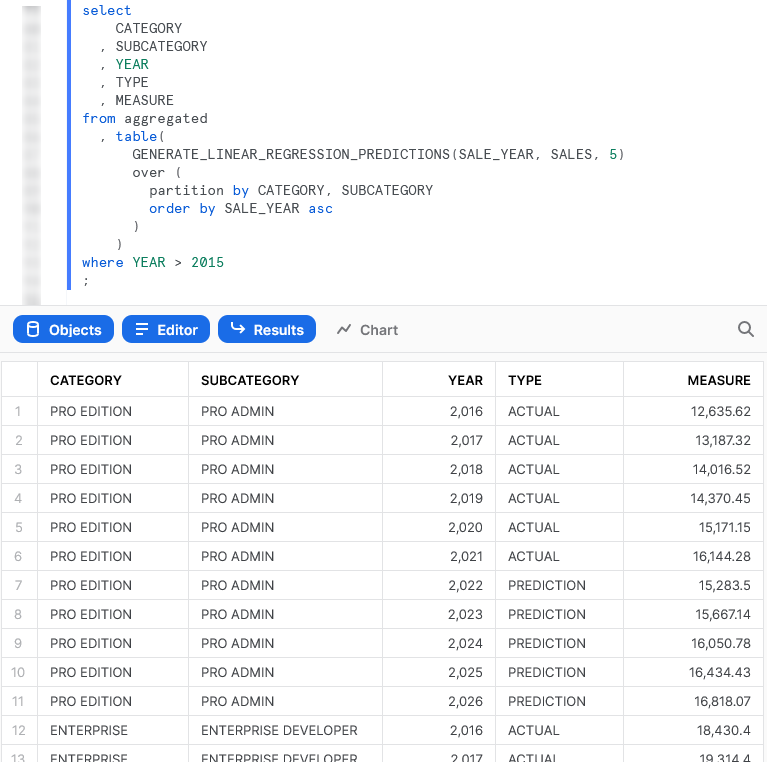## Importing Files and Libraries via a Stage

So far, we have demonstrated quite a lot in our miniature crash course into Python UDTFs in Snowflake. Before we wrap up, I’d like to cover one last piece, which is how to import libraries that are not covered by the Anaconda Snowflake channel and how to import other external files.

### Warning Regarding Staged Files and Libraries

It is important to note at this time that these external files are fixed in time. UDTFs do not have access to the “outside world,” and each file must exist within the stage at the time the UDTF is created as the file is actually copied into Snowflake’s underlying storage location for the UDTF. If you update your files in your external stage, your Snowflake UDTF will not undergo these changes unless you recreate the UDTF, as well.

Unfortunately, files must be imported into UDTFs individually and specifically. If you wish to import five files within a directory, you must list all five files individually. You cannot simply specify a parent directory or leverage a wildcard.

### Examples Using External Files and Libraries

For these examples, we will be uploading all of our required files to the stage “STG_FILES_FOR_UDTFS.”

#### Import an External Excel xlsx File

This example reads an Excel xlsx file into a pandas dataframe then uses information in that file to map the input string to the corresponding output.

The file we will be leveraging is a very simple mapping file that looks like this: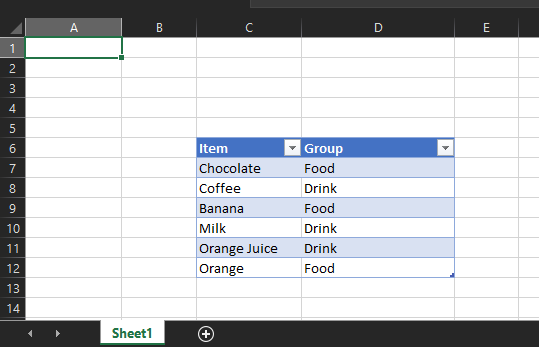The file is called “Dummy Mapping File.xlsx,” and I have uploaded it to the stage “STG_FILES_FOR_UDTFS.”

Here is the code for the UDTF itself:

##################################################################
## Define the class for the UDTF

# Import the required modules
import pandas
import sys

# Spoof sys._xoptions so that class can be created
sys._xoptions["snowflake_import_directory"] = 'Supporting Files/'

# Define handler class
class leverage_external_mapping_file :

## Retrieve the Snowflake import directory
IMPORT_DIRECTORY_NAME = "snowflake_import_directory"
import_dir = sys._xoptions[IMPORT_DIRECTORY_NAME]

## Ingest the mapping into a Pandas dataframe
df_mapping = pandas.read_excel(import_dir + 'Dummy Mapping File.xlsx', skiprows=5, usecols="C:D")

## Define process method that acts
## on each individual input row
def process(
self
, input_item: str
) :

### Apply the mapping to retrieve the mapped value
df_mapped_group = self.df_mapping[self.df_mapping['Item']==input_item]

mapped_group = 'No matching group found'

if len(df_mapped_group.index) > 0 :
mapped_group = df_mapped_group.iloc['Group']

yield(mapped_group,)

##################################################################
## Register UDTF in Snowflake

### Add packages and data types
from snowflake.snowpark.types import StructType, StructField
from snowflake.snowpark.types import StringType

### Define output schema
output_schema = StructType([
StructField("MAPPED_ITEM", StringType())
])

snowpark_session.udtf.register(
handler = leverage_external_mapping_file
, output_schema = output_schema
, input_types = [StringType()]
, is_permanent = True
, name = 'SNOWPARK_LEVERAGE_EXTERNAL_MAPPING_FILE'
, replace = True
, stage_location = '@UDTF_STAGE'
)

The unique differences for this example are:

• Row 45 includes a line to import the file from a local repository. This is a critical component for our Python function to be able to access the file during the pandas.from_excel() method.
• Mirroring this is the code on lines 15-16 that leverages the sys library to access the location where Snowflake stores files that have been imported into the UDTF. The file is then ingested using pandas on row 19.
• On row 29, we leverage the imported dataframe. As we have defined it within a class, we leverage self. to retrieve the variable from the class.
• Some strange activity occurs on row 9 where we define a value for sys._xoptions["snowflake_import_directory"]. This value will not be leveraged in the UDTF once it is registered to Snowflake; however, we must define it so that we can define the class locally without errors. Note that this is the directory that contains the local copy of the file we wish to import on row 45.

The rest of the code in this script is specific Python code to download the Excel file into a dataframe, filter it to our specific item and return the matched group value.

Again, if we execute this code as part of a Python script, we can then call the table function using the snowpark_session.sql().show() method to see the result. This screenshot omits the code itself for brevity.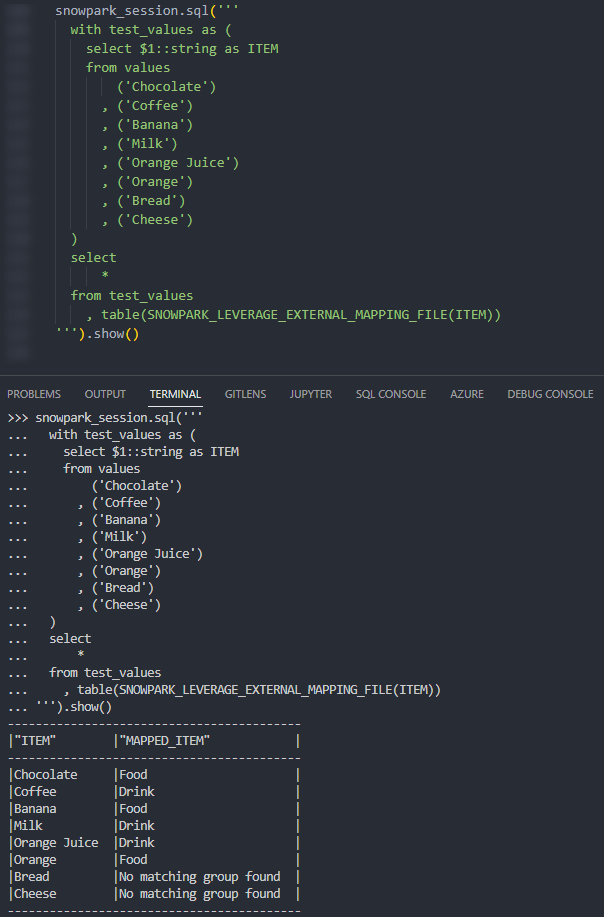Again, we can also call the table function from within a Snowflake worksheet whilst specifying our desired partitions to see the result.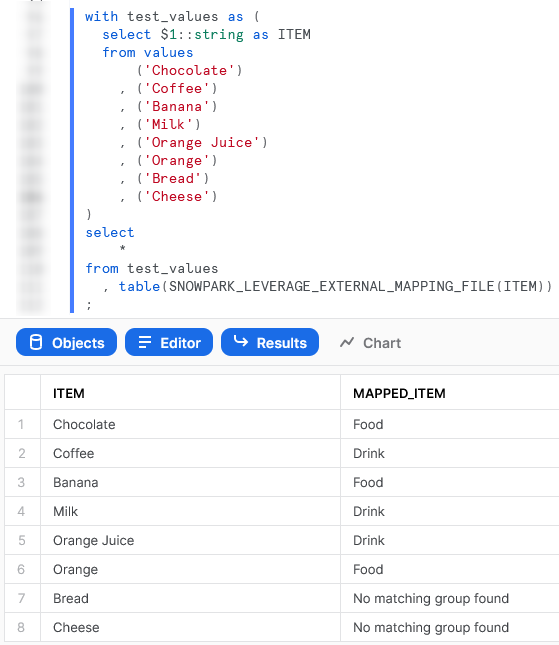#### Import a Non-Standard External Library

This example is designed to leverage the xldate function of the xlrd library. This library is not included in the standard set of supported libraries, so it must be imported specifically.

Let’s see the code:

##################################################################
## Define the class for the UDTF

# Import the required modules
import sys

# Spoof sys._xoptions so that class can be created
sys._xoptions["snowflake_import_directory"] = 'Supporting Files/xlrd/'

# Define handler class
class leverage_external_library :

## Retrieve the Snowflake import directory
IMPORT_DIRECTORY_NAME = "snowflake_import_directory"
import_dir = sys._xoptions[IMPORT_DIRECTORY_NAME]

## Import the required external modules using the importlib.util library
import importlib.util
module_spec = importlib.util.spec_from_file_location('xldate', import_dir + 'xldate.py')
xldate = importlib.util.module_from_spec(module_spec)

## Define process method that acts
## on each individual input row
def process(
self
, input_int: int
) :

### Apply the mapping to retrieve the mapped value
xl_datetime = self.xldate.xldate_as_datetime(input_int, 0)

yield(xl_datetime,)

##################################################################
## Register UDTF in Snowflake

### Add packages and data types
from snowflake.snowpark.types import StructType, StructField
from snowflake.snowpark.types import TimestampType, IntegerType

### Define output schema
output_schema = StructType([
StructField("EXCEL_TIMESTAMP", TimestampType())
])

snowpark_session.udtf.register(
handler = leverage_external_library
, output_schema = output_schema
, input_types = [IntegerType()]
, is_permanent = True
, name = 'SNOWPARK_LEVERAGE_EXTERNAL_LIBRARY'
, replace = True
, stage_location = '@UDTF_STAGE'
)

The unique differences for this example are:

• Row 42 includes a line to import the entire xlrd subdirectory from a local repository. This is critical, and would match the file found in your env/Lib/site-packages/xlrd directory that is created during a pip install in a virtual environment (or your default environment).
• As with the previous example, lines 14-15 leverage the sys library to access the location where Snowflake stores files that have been imported into the UDTF.
• The block of lines 18-21 leverage the importlib.util library to import the specific module from the imported directory. This imported library is then leveraged on row 31. As we have defined it within a class, we leverage self. to retrieve the imported library.
• Again, on row 8 we define a value for sys._xoptions["snowflake_import_directory"]. This value will not be leveraged in the UDTF once it is registered to Snowflake; however, we must define it so that we can define the class locally without errors. Note that this is the directory that contains the local copy of the xldate.py file, which is one of the files within the subdirectory that is imported on row 42.

Again, if we execute this code as part of a Python script, we can then call the table function using the snowpark_session.sql().show() method to see the result. This screenshot omits the code itself for brevity. In this example, the test values are created using Snowflake’s generator function.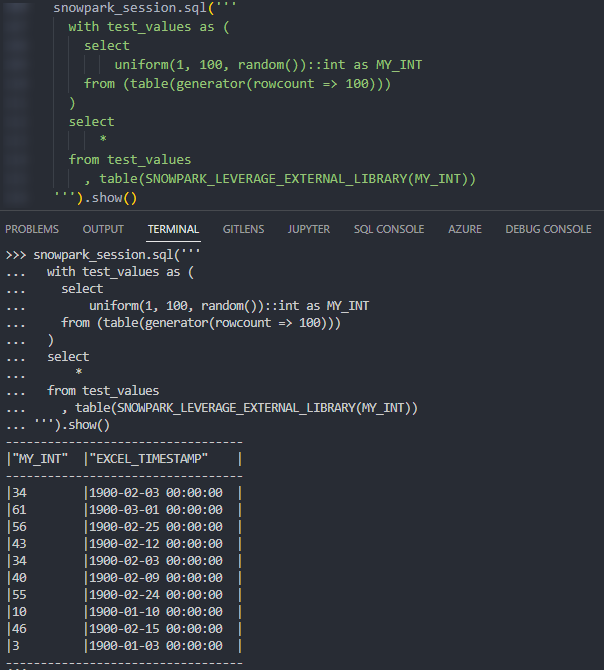Again, we can also call the table function from within a Snowflake worksheet whilst specifying our desired partitions to see the result.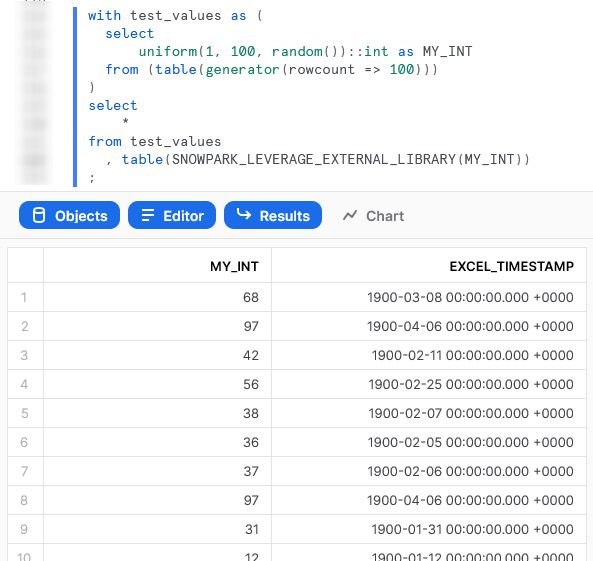## Wrap Up

So there we have it. We have covered a lot of content in this post and some of these UDTFs could potentially have their own blog post all on their own! My goal is to demonstrate how to create UDTFs for different scenarios and provide that in a single post so that it is easy for readers to find and refer back to if needed.

I hope this helps you get started on your own journey with Python UDTFs in Snowflake using Snowpark. Let us know what cool things you come up, it’s always great to see new functionality lead to productive and useful innovation.

If you wish to see the code for all of this, and other content that we have, you can find it on the InterWorks GitHub.

If you are interested in finding out about Python UDFs or stored procedures instead of UDTFs, or wish to achieve this through the UI, be sure to check out my other Definitive Guides for Snowflake with Python.

### The Latest

• ##### The 16 Greatest Words in the English Language and How They Relate to Data

Configure Azure Private Link Connectivity with Snowflake Snowflake and Azure are incredibly capable of storing data securely. You can see all reports available to customers through their pages ...
Matillion Visionary 2023 Emerald Award | NHS Greater Manchester | InterWorks InterWorks were delighted to hear that NHS Greater Manchester Integrated Care has earned a Matillion Emerald Award as a Visionary in ...

See more from this author →

InterWorks uses cookies to allow us to better understand how the site is used. By continuing to use this site, you consent to this policy. Review Policy OK

×

Interworks GmbH
Ratinger Straße 9
40213 Düsseldorf
Germany
Geschäftsführer: Mel Stephenson

Kontaktaufnahme: markus@interworks.eu
Telefon: +49 (0)211 5408 5301

Amtsgericht Düsseldorf HRB 79752
UstldNr: DE 313 353 072

×